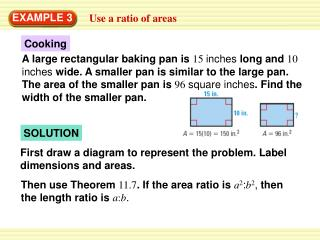# EXAMPLE 3 - PowerPoint PPT PresentationDownload PresentationEXAMPLE 3

Download Presentation## EXAMPLE 3

- - - - - - - - - - - - - - - - - - - - - - - - - - - E N D - - - - - - - - - - - - - - - - - - - - - - - - - - -
##### Presentation Transcript

1. A large rectangular baking pan is 15 inches long and 10inches wide. A smaller pan is similar to the large pan. The area of the smaller pan is 96square inches. Find the width of the smaller pan. EXAMPLE 3 Use a ratio of areas Cooking SOLUTION First draw a diagram to represent the problem. Label dimensions and areas. Then use Theorem 11.7. If the area ratio is a2:b2, then the length ratio is a:b.

2. Area of smaller pan 96 16 = = 25 150 Area of large pan 4 Length in smaller pan = 5 Length in large pan ANSWER 4 Any length in the smaller pan is , or 0.8, of the corresponding length in the large pan. So, the width of the smaller pan is 0.8(10 inches)8 inches. 5 = EXAMPLE 3 Use a ratio of areas Write ratio of known areas. Then simplify. Find square root of area ratio.

3. Gazebo EXAMPLE 4 Solve a multi-step problem The floor of the gazebo shown is a regular octagon. Each side of the floor is 8 feet, and the area is about 309square feet.You build a small model gazebo in the shape of a regular octagon. The perimeter of the floor of the model gazebo is 24inches. Find the area of the floor of the model gazebo to the nearest tenth of a square inch.

4. 64 ft Perimeter of full-sized 32 8(8 ft) = = = 1 24 in. Perimeter of model 2 ft EXAMPLE 4 Solve a multi-step problem SOLUTION All regular octagons are similar, so the floor of the model is similar to the floor of the full-sized gazebo. Find the ratio of the lengths of the two floors by finding the ratio of the perimeters. Use the same units for both lengths in the ratio. STEP 1 So, the ratio of corresponding lengths (full-sized to model) is 32:1.

5. Area of full-sized (length of full-sized)2 = Area of model (length of model)2 322 309 ft2 = x ft2 12 1024x 309 = EXAMPLE 4 Solve a multi-step problem STEP 2 Calculate the area of the model gazebo’s floor. Let xbe this area. Theorem11.7 Substitute. Cross Products Property x ≈0.302 ft2 Solve for x.

6. 0.302 ft2 144in.2 ≈ 43.5in.2 1ft.2 ANSWER The area of the floor of the model gazebo is about 43.5square inches. EXAMPLE 4 Solve a multi-step problem STEP 3 Convert the area to square inches.

7. Area of smaller decagon 20 5 = = 9 36 Area of large decagon √ 5 Length in smaller decagon = 3 Length in large decagon for Examples 3 and 4 GUIDED PRACTICE 2. The ratio of the areas of two regular decagons is 20:36. What is the ratio of their corresponding side lengths in simplest radical form? SOLUTION Then use Theorem 11.7. If the area ratio is a2:b2, then the length ratio is a:b. Write ratio of known areas. Then simplify. Find square root of area as ratios.

8. = 2 35 + 2 20 for Examples 3 and 4 GUIDED PRACTICE 3. Rectangles I and II are similar. The perimeter of Rectangle I is 66 inches. Rectangle II is 35 feet long and 20 feet wide. Show the steps you would use to find the ratio of the areas and then find the area of Rectangle I. SOLUTION Perimeter of Rectangle I = 66 inches Perimeter of Rectangle II = 2(l + b) = 2(35 + 20) = 110 feet.

9. = 110 12 1 66 Perimeter of Rectangle I = = 20 1320 Perimeter of Rectangle II for Examples 3 and 4 GUIDED PRACTICE Convert110 feet to inches. 110 ft = 1320 inches. Find the ratio of the lengths of the two rectangles by finding the ratio of the perimeters. STEP 1 So, the ratio of the corresponding lengths to is 1:20.

10. = 700 144 (sides of RectangleI)2 Area of RectangleI = (sides of RectangleII)2 Area of RectangleII 1 1 = 202 400 for Examples 3 and 4 GUIDED PRACTICE STEP 2 Find the area of Rectangle II. Area of Rectangle II = lb = 35(20) = 700 feet2 Convert700 feet2to inches2. 700 ft2 = 100,800 inches2. STEP 3 Find the ratio of their Areas

11. Area of Rectangle I = 100,800 · 1 400 for Examples 3 and 4 GUIDED PRACTICE STEP 4 Find the area of Rectangle I. Area of Rectangle I = Area of Rectangle II · (ratio of areas) = 252 in.2